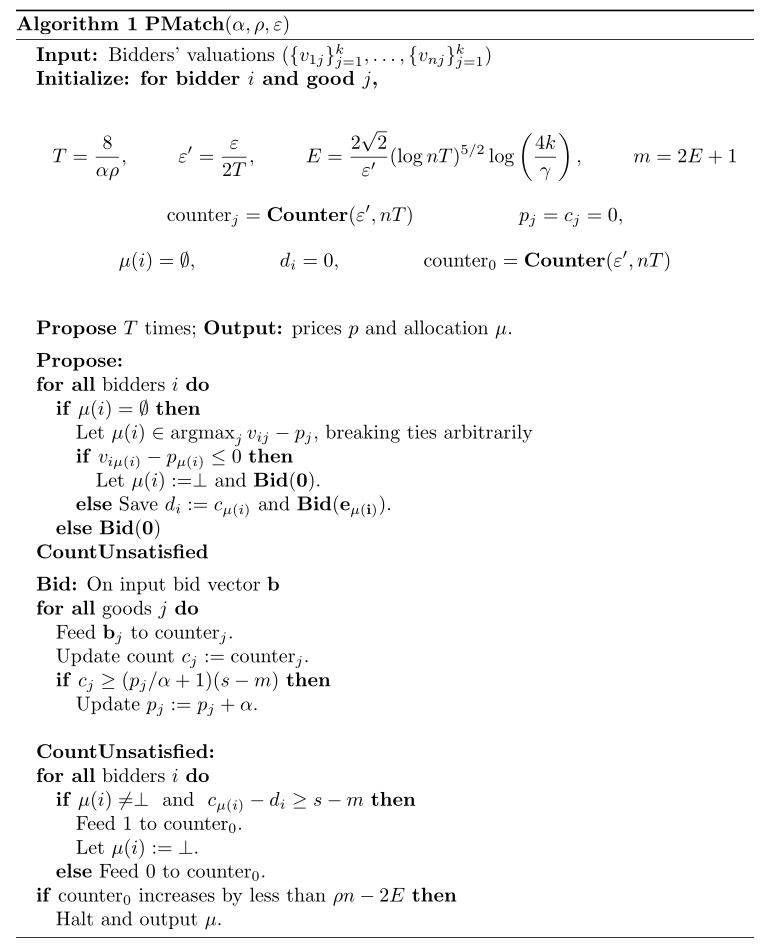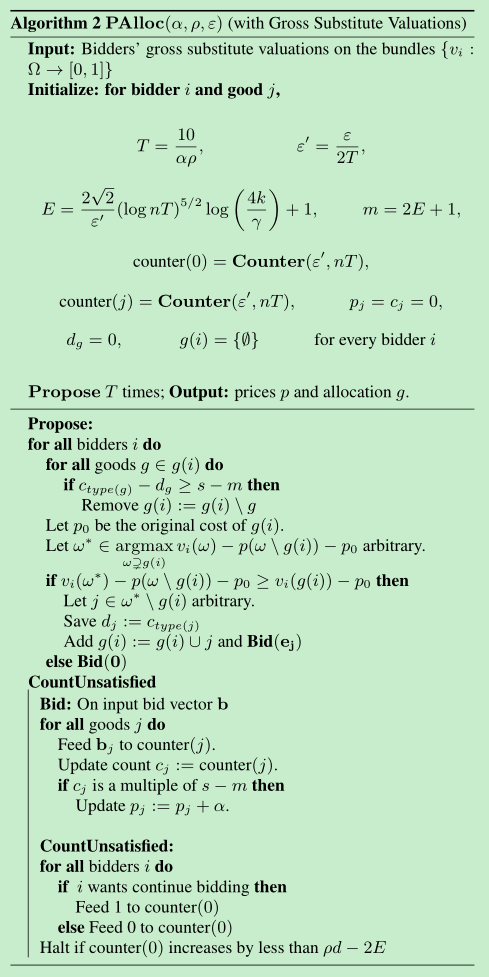Justin Hsu, et al. Private matchings and allocations. SIAM Journal on Computing, 2016.

Justin Hsu, Zhiyi Huang, Aaron Roth, Tim Roughgarden, and Zhiwei Steven Wu. Private matchings and allocations. STOC 2014.

• Differential Privacy & Resource Allocation

## 文章概要

• $k$ goods：$j\in \{1,\ldots,k\}$$n$ buyers：$i\in \{1,\ldots,n\}$;
• Each $i$ has a value $v_{ij}\in[0,1]$ for each good $j$.

Our goal 找到 buyer-good 匹配 $\mu$，s.t. 最大化社会总福利 $SW=\sum\limits_{i=1}^nv_{i,\mu(i)}$.

• 隐私性问题：values 是敏感信息，会涉及隐私.

### DP在此问题上的局限性

It is not hard to see that this goal is impossible under the standard notion of differential privacy.

• 解决方法：使用 Kearns 等在 2014 年提出的联合差分隐私（Joint DP）

### 思路：将 Market Clearing prices 与 DP 结合

• 使用 Walrasian equilibrium prices：
• 在 Joint DP 的要求下计算 Walrasian equilibrium prices.
• Kelso 和 Crawford 在 1982 的经典分析：用递增价格拍卖来计算 Walrasian…

## 基础知识

• 物品集 $G$，以及 agent 集合 $[n]$
• 每个 agent $i\in[n]$ 都有一个估值函数 $v_i:2^G\to [0,1]$
• 一个可行的分配是一列集合 $S_1,\ldots,s_n\subseteq G$，s.t. $S_i\cap S_j=\varnothing$$\forall i\neq j$.
• 上述分配的社会总福利为 $\sum\limits_{i=1}^n v_i(S_i)$.
• 最大社会总福利 $OPT=\max\limits_{S_1,\ldots,S_n}\sum\limits_{i=1}^n v_i(S_i)$.

• 但本文的结果对于 gross substitute valuation 也同样成立(简言之，就是物品 $i$ 涨价会使得其他物品更受欢迎，参见 wiki: Gross substitutes).

### 形式化描述

• 价格向量 $\{p_g\}_{g\in G}$，物品集 $S$ 对参与者 $i$ 的效用为 $u_i(S,p)=v_i(S)-\sum\limits_{j\in S}p_j$.
• 若定价 $p$$p'$ 满足 $p_g\leqslant p'_g$$\forall g\in G$，则记为 $p \preceq p^{\prime}$.
• 在定价 $p$ 下，参与者 $i\in[n]$ 最想要的物品集为 $\omega(p)=\arg \max\limits _{S \subseteq G} u_{i}(S, p)$.

Def 现有估值函数 $v_i: 2^G\to [0,1]$，如果对于定价向量 $p\preceq p'$ 以及 $\forall S\in\omega(p)$，若 $S'\subseteq S$ 满足 $p'_g=p_g$$\forall g\in S'$，则存在 $S^*\in\omega(p)$，s.t. $S'\subset S^*$，则称 $v_i$ 满足 gross substitutes.

• 这个定义很别扭…我没懂
• 本文的工作还是只考虑了一人一个物品的情况.

• 设共有 $k$ 种物品，代表元为 $g_1,\ldots,g_k\in G$
• 若所有人都认为两个物品 $g_1,g_2\in G$ 等值，则这两个物品就被认为是相同的.

### 差分隐私

Def of DP 若算法 $\mathcal{M}: C^{n} \rightarrow \mathcal{R}$ 满足 $\forall D,D'\in C^n$，s.t. $|D\triangle D'|=1$，以及 $\forall S\subseteq \mathcal{R}$，总有$\operatorname{Pr}[\mathcal{M}(D) \in S] \leq e^{\epsilon} \operatorname{Pr}\left[\mathcal{M}\left(D^{\prime}\right) \in S\right]+\delta$则称 $\mathcal{M}$$(\epsilon,\delta)$-差分隐私的. 若 $\delta=0$，则称为 $\epsilon$-差分隐私.

• 由于在资源分配问题中，输出结果是 $n$ 元集列，因而 $\mathcal{M}$ 的陪域为 $(2^G)^n$.

Joint DP 若算法 $\mathcal{M}: C^n\to (2^G)^n$ 满足：对 $\forall i$ 以及 $i$-neighbors $D,D'\in C^n$，以及 $S\subseteq (2^G)^n$，总有： $\operatorname{Pr}\left[\mathcal{M}(D)_{-i} \in S\right] \leq e^{\epsilon} \operatorname{Pr}\left[\mathcal{M}\left(D^{\prime}\right)_{-i} \in S\right]+\delta$ 则称其满足 $(\epsilon,\delta)$-联合差分隐私.

### DP Counter

• 一个二进制串 $\sigma=(\sigma_1,\ldots,\sigma_T)\in\{0,1\}^T$，计数器 $\mathcal{M}$ 给出了 $c_\sigma(t)=\sum\limits_{i=1}^t \sigma_i$ 的一个近似.

### 隐私化的最大匹配

• $n$ 个人，$k$ 种物品，每种 $s$ 个. 估值函数 $v_{ij}\in[0,1]$
• 定价向量 $p\in[0,1]^k$，分配方案 $\mu: [n]\to[k]\cup\{\perp\}$
• $\perp$ 的解释：未匹配到物品的人被统一匹配到一个虚拟物品，记作 $\perp$.

Def 给定定价向量 $p\in[0,1]^k$ 以及从人到物的匹配 $\mu:[n]\to[k]\cup\{\perp\}$，若 $\mu$ 满足：

• 至少 $(1-\rho)$ 比例的人匹配到的物品是 $\alpha$-最优的； - 即对至少 $(1-\rho)$ 比例的 $i$ 而言，有 $v_{i \mu(i)}-p_{\mu(i)} \geq v_{i j}-p_{j}-\alpha$$\forall j$.
• 每种物品被分配的量不能超过总供应量；
• 每种供不应求的物品(over-demanded)都会被抢光，除非其供应量不超过 $\beta$. - 原文：each over-demanded good clears except for at most β supply.

#### 算法描述：PMatch Algorithm

Propose Function

• 同时进行 $k$ 场竞价选举，每场代表一种物品.
• 任意时刻，每种物品的 proposal price 为 $p_j$.
• 给所有参与者规定一个（大家都知道的）顺序，依次访问每一个人.
• 给访问到的未被匹配到物品的参与者分配一个对他而言效用最大的物品.

Bid Function

• 对每类物品来说：申请与某类物品匹配的前 $s$ 名暂时获得该类物品
• 对某物品而言，若某个人比其当前持有者出价更高，则该物品将易主.
• 对每个物品的出价每次上涨固定值 $\alpha$.这篇文章使用的是所谓“广告牌模型”（Billboard Model）

1. 可能有 $\rho$ 比例的人在算法结束时仍处于未匹配状态；
2. 被匹配了物品的人也未必得到了自己最想要的的物品；
3. 整个拍卖过程会分出一部分物品来应对 Counters 中的噪音可能带来的过量分配. 而这些物品有可能会卖不出去.

## 推广